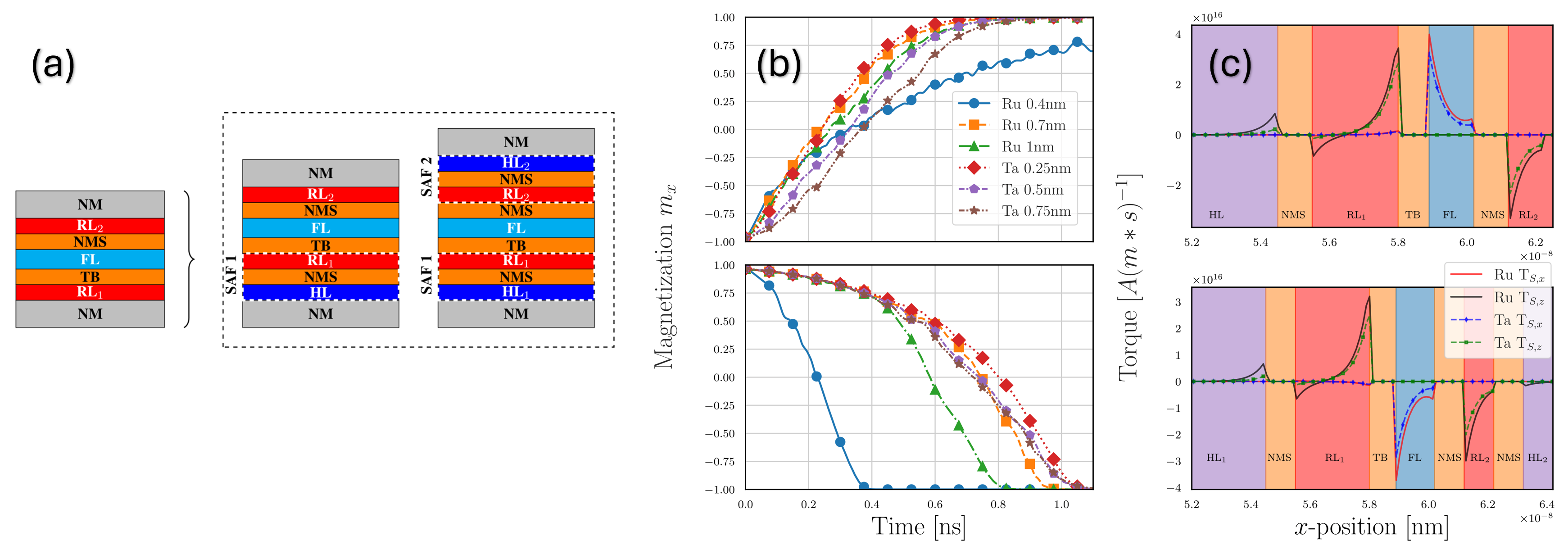Mario Bendra Dipl.-Ing. PublicationsBiography

Mario Bendra was born in 1993 in Steyr, Austria. He received his BSc degree in Mechanical Engineering from the University of Applied Sciences Upper Austria in 2017. After an exchange semester at the University of the Sunshine Coast, Australia in 2018, he received the degree of Diplomingenieur in Mechanical Engineering from the University of Applied Sciences Upper Austria in 2019. Mario joined the Institute for Microelectronics in October 2020, where he is working towards his doctoral degree focusing on developing and implementing advanced computational approaches to simulate and optimize spin-transfer torque magnetoresistive memories.

A Finite Element Method Approach to MRAM Modeling: Verification and Calibration

The Landau-Lifschitz-Gilbert (LLG) equation describes the magnetization dynamics of a magnetoresistive RAM (MRAM) in an effective magnetic field. As we are dealing with spin-transfer torque MRAM (STT-MRAM), the LLG equation has to be supplemented with an additional torque term. The torque acting on the magnetization is created by the nonequilibrium spin accumulation caused by the electrical current running through the structure. A typical spin-transfer torque MRAM bit cell consists of a magnetic tunnel junction connected between two electrodes, as shown in Fig. 1.

The partial differential LLG equation, with an additional Slonczewski-like torque, is solved via the finite element method. To enable verification and comparison of the finite element method, the more straightforward finite difference method is used as a reference.

In order to compare the two methods and to improve and control the accuracy of the evaluation of the magnetization dynamics, different time integration schemes for the finite element method are implemented. Since the LLG equation is a stiff partial differential equation, it is desirable to use implicit time integration schemes.

In addition to the Crank-Nicholson-like time integration scheme currently in use, we introduce four different methods that can all be derived from the general implicit Runge-Kutta equation using correct Butcher tables. The schemes used are Backward Euler, Midpoint Scheme, two-stage Singly Diagonal Implicit Runge-Kutta (SDIRK23) and the three-stage Singly Diagonal Implicit Runge-Kutta (SDIRK34). In Fig. 2, the simplest method, the Backward Euler, is displayed. The abscissa shows the time in seconds and the ordinate shows the magnetization in the z-direction, which corresponds to the rotation axis of the spin-transfer torque MRAM bit cell. Furthermore, one can see that the time step sizes 0.2e^(−12) and 0.2e^(−1) present similar accuracies.

Due to the introduction of more precise methods, we have succeeded in increasing the time step size by two orders of magnitude while reducing the calculation time considerably.Fig. 1: Spin-transfer torque MRAM bit cell.Fig. 2: Backward Euler method with variable time step size.